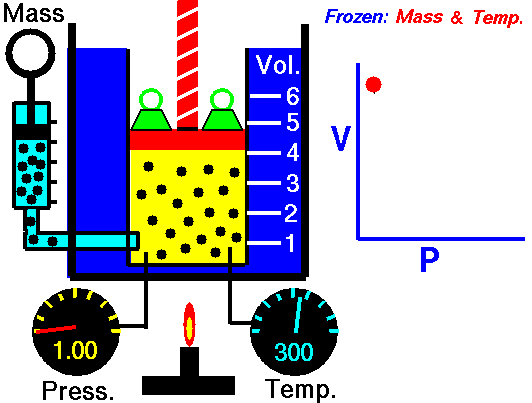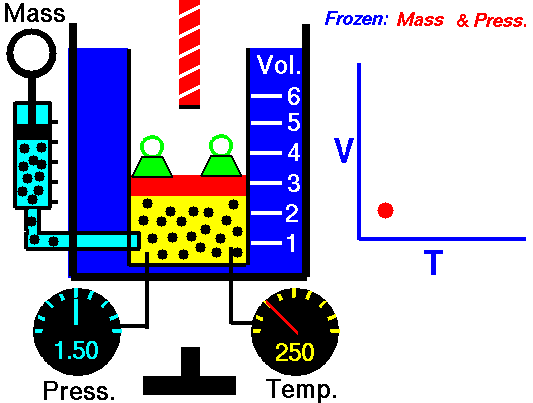It looks like you're using Internet Explorer 11 or older. This website works best with modern browsers such as the latest versions of Chrome, Firefox, Safari, and Edge. If you continue with this browser, you may see unexpected results.

# Chemistry

Properties of Gases:

• Compressible
• Expands or shrinks as the temperature increases or decreases
• Low density
• Miscible with other gases

Pressure in general is defined as force per unit area.

Gas pressure: Gas pressure in a container is the amount of force exerted per unit area by the moving gas molecules due to their collision with the surface wall. Pressure increases or decreases depending on the gas temperature, the number of molecules present, and the volume of the container.

 Pressure Units pascal (Pa). 1 Pa = 1 N/m2 (recommended IUPAC unit) kilopascal (kPa). 1 kPa = 1000 Pa pounds per square inch (psi). air pressure at sea level is ~14.7 psi atmosphere (atm). 1 atm = 101,325 Pa = 760 torr air pressure at sea level is ~1 atm bar (bar, or b) 1 bar = 100,000 Pa (exactly) millibar (mbar, or mb). 1000 mbar = 1 bar inches of mercury (in. Hg). 1 in. Hg = 3386 Pa torr 1 torr = 1/760 atm millimeters of mercury (mm Hg). 1 mm Hg ~1 torr

Relating Pressure, Volume, Amount, and Temperature: The Ideal Gas Law

The relationships between the various macroscopic physical properties of gases - pressure, volume, number of molecules, and temperature - are established using the ideal gas law.

The ideal gas law is expressed using the following formula:

PV = nRT

Where :

P = pressure

V = volume

n = number of molecules

R = gas constant

T = temperature

Boyle's Law

This law demonstrates that the pressure of a gas is inversely proportional to the volume when other variables (such as the number of molecules and temperature) are kept constant. In other words, as the volume increases, the pressure decreases - and vice versa - as shown in the animation below (the green blocks in the animation represent pressure).Figure 1: Animated Boyle's Law. From Tom Benson (2015, May 5) for the Glenn Research Center, NASA. https://www.grc.nasa.gov/WWW/K-12/airplane/aboyle.html

Boyle's Law Formula

PiVi = PfVf

Where :

Pi = initial pressure

Vi = initial volume

Pf = final pressure

Vf = final volume

Charles' Law

Charles' law demonstrates that when the pressure of a dry gas sample is kept constant, its volume is directly proportional to its temperature (in Kelvin) as shown in the animation below (the green blocks in the animation represent the pressure).Figure 2: Animated Charles and Gay-Lussac's Law. From Tom Benson (2015, May 5) for the Glenn Research Center, NASA. https://www.grc.nasa.gov/www/k-12/airplane/aglussac.html

Charles' Law FormulaWhere :

Ti = initial temperature

Vi = initial volume

Tf = final temperature

Vf = final volume

Combined Gas Law

Boyle's law and Charles' law are combined into form combined gas law as shown below.